Learn All Concepts of Chapter 3 Class 12 Matrices - FREE. Check - Matrices Class 12 - Full video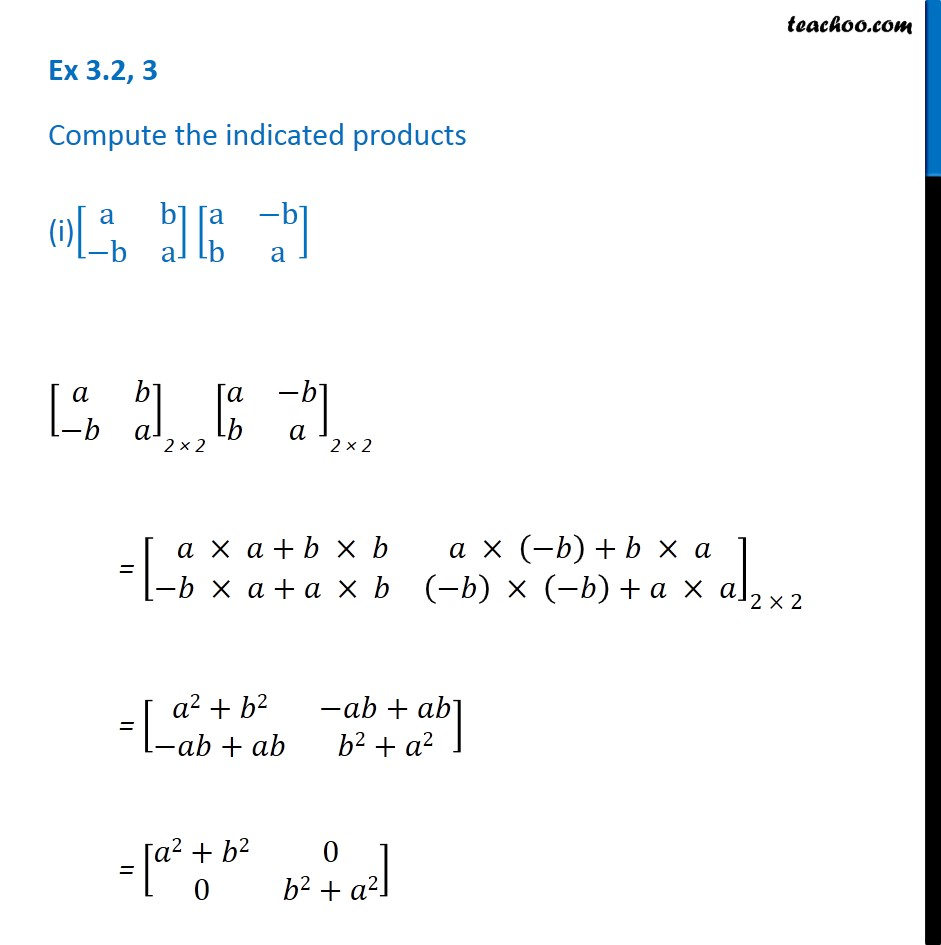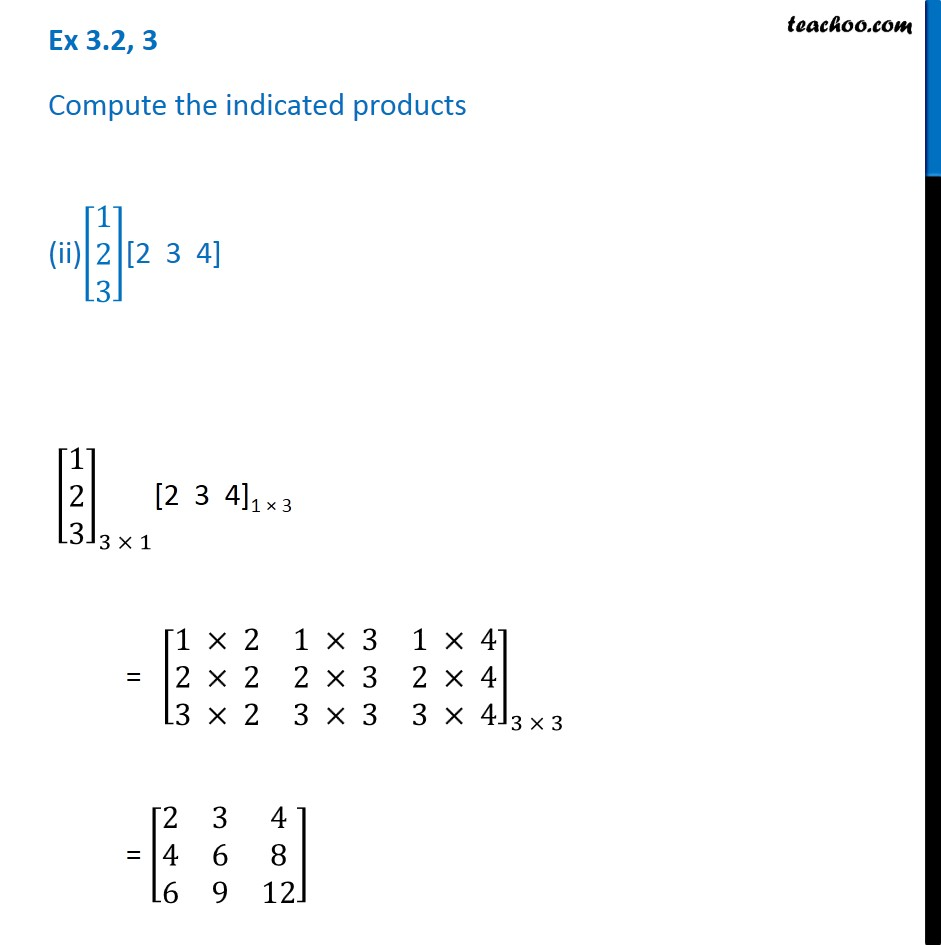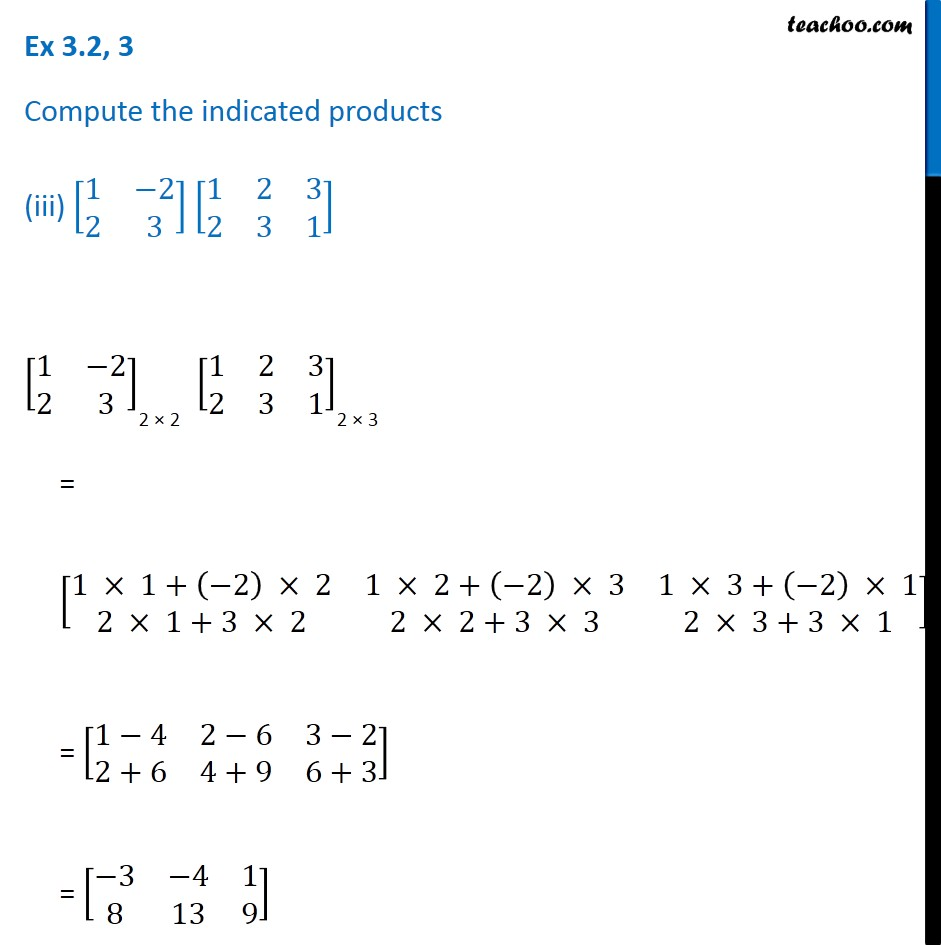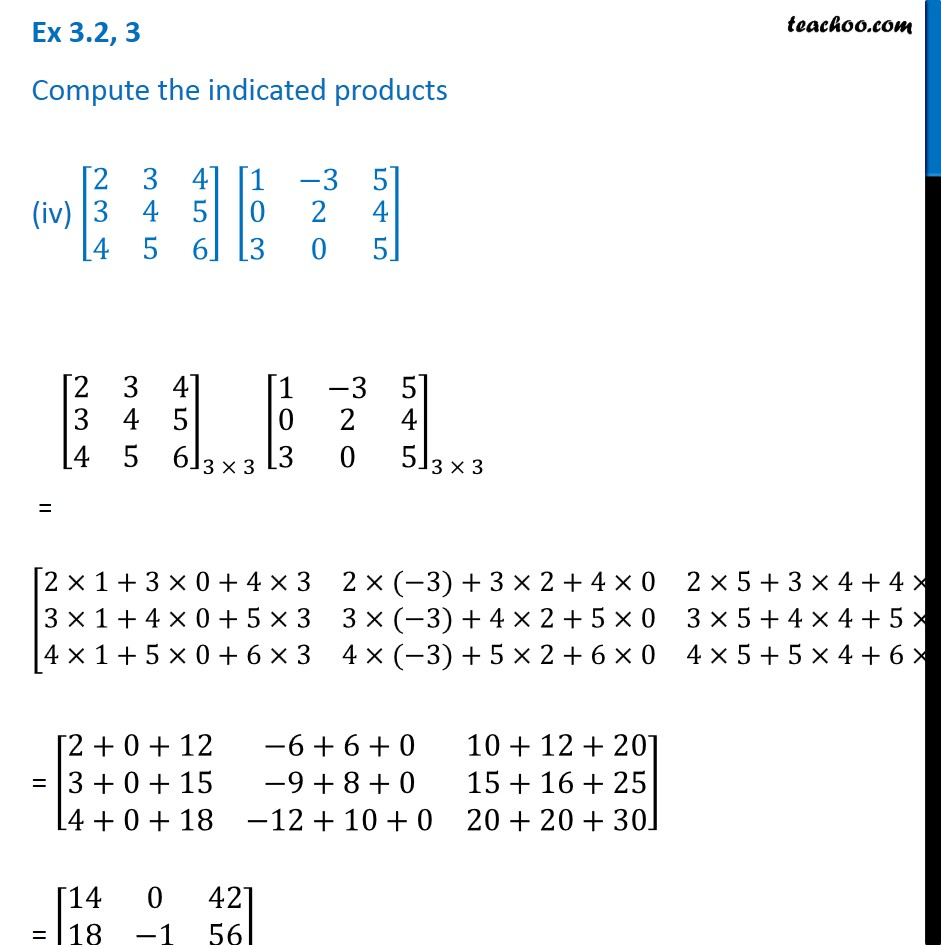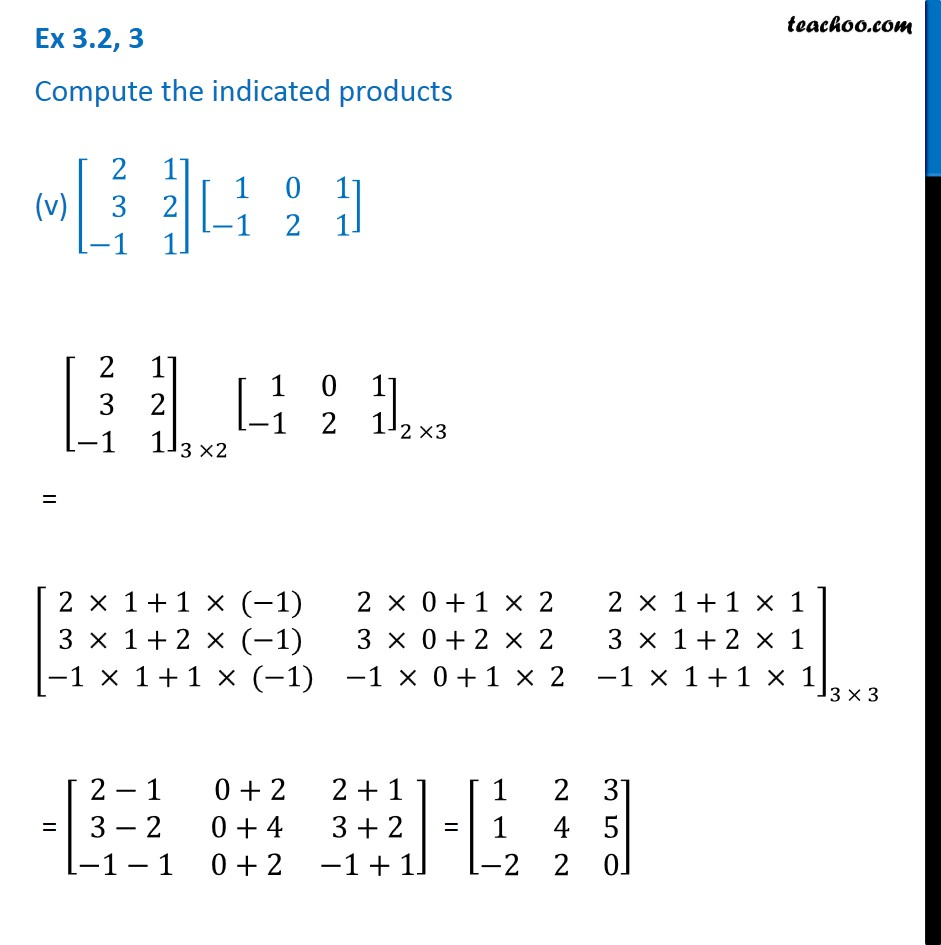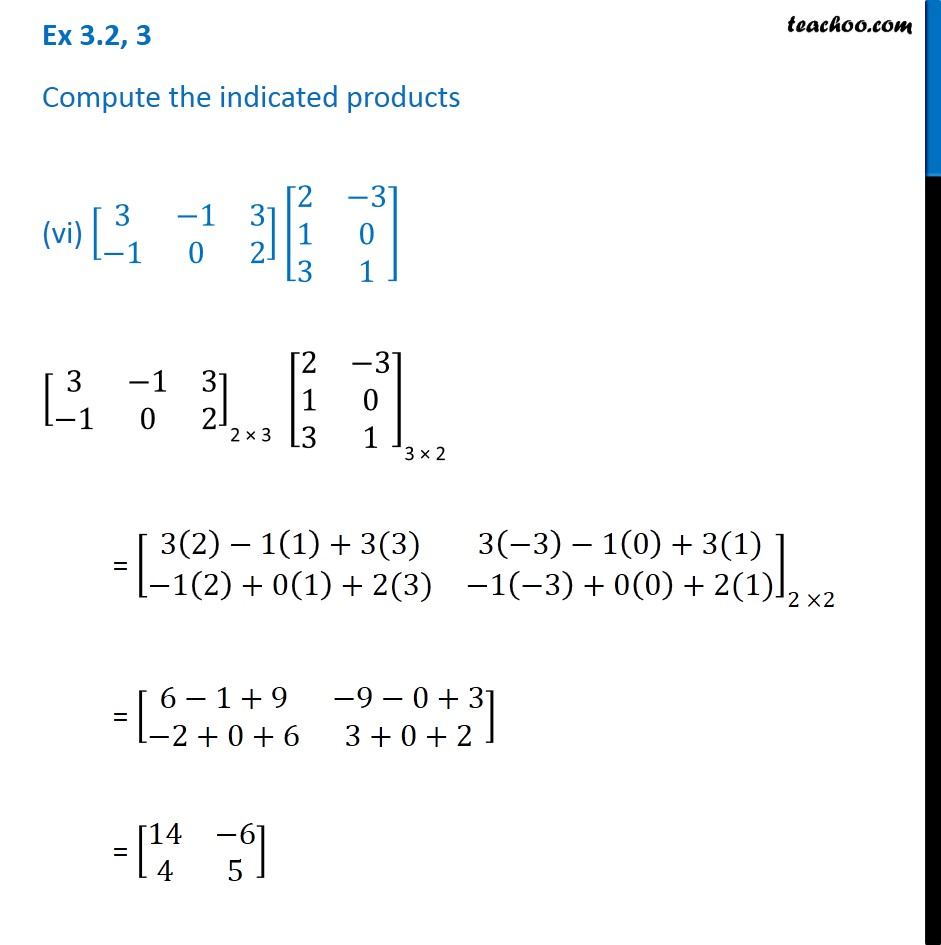1. Chapter 3 Class 12 Matrices
2. Concept wise
3. Multiplication of matrices

Transcript

Ex 3.2, 3 Compute the indicated products (i)[■8(a&b@−b&a)] [■8(a&−b@b&a)] [■8(𝑎&𝑏@−𝑏&𝑎)]_"2 × 2" [■8(𝑎&−𝑏@𝑏&𝑎)]_"2 × 2" = [■8( 𝑎 × 𝑎+𝑏 × 𝑏&𝑎 × (−𝑏)+𝑏 × 𝑎@−𝑏 × 𝑎+𝑎 × 𝑏&(−𝑏) × (−𝑏)+𝑎 × 𝑎)]_(2 × 2) = [■8(𝑎2+𝑏2&−𝑎𝑏+𝑎𝑏@−𝑎𝑏+𝑎𝑏&𝑏2+𝑎2)] = [■8(𝑎2+𝑏2&0@0&𝑏2+𝑎2)] Ex 3.2, 3 Compute the indicated products (ii)[█(1@2@3)][2 3 4] [█(1@2@3)]_(3 × 1)[2 3 4]1 × 3 = 〖" " [■8(1 × 2&1 × 3&1 × 4@2 × 2&2 × 3&2 × 4@3 × 2&3 × 3&3 × 4)]〗_(3 × 3) = [■8(2&3&4@4&6&8@6&9&12)] Ex 3.2, 3 Compute the indicated products (iii) [■8(1&−2@2&3)] [■8(1&2&3@2&3&1)] [■8(1&−2@2&3)]_"2 × 2" [■8(1&2&3@2&3&1)]_"2 × 3" = [■8(1 × 1+(−2) × 2&1 × 2+(−2) × 3&1 × 3+(−2) × 1@2 × 1+3 × 2&2 × 2+3 × 3&2 × 3+3 × 1)]_(2 × 3) = [■8(1−4&2−6&3−2@2+6&4+9&6+3)] = [■8(−3&−4&1@8&13&9)] Ex 3.2, 3 Compute the indicated products (iv) [■8(2&3&4@█(3@4)&█(4@5)&█(5@6))] [■8(1&−3&5@█(0@3)&█(2@0)&█(4@5))] [■8(2&3&4@█(3@4)&█(4@5)&█(5@6))]_(3 × 3) [■8(1&−3&5@█(0@3)&█(2@0)&█(4@5))]_(3 × 3) = [■8(2×1+3×0+4×3&2×(−3)+3×2+4×0&2×5+3×4+4×5@3×1+4×0+5×3&3×(−3)+4×2+5×0&3×5+4×4+5×5@4×1+5×0+6×3&4×(−3)+5×2+6×0&4×5+5×4+6×5)]_(3 × 3) = [■8(2+0+12&−6+6+0&10+12+20@3+0+15&−9+8+0&15+16+25@4+0+18&−12+10+0&20+20+30)] = [■8(14&0&42@18&−1&56@22&−2&70)] Ex 3.2, 3 Compute the indicated products (v) [■8( 2&1@ 3&2@−1&1)] [■8( 1&0&1@−1&2&1)] [■8( 2&1@ 3&2@−1&1)]_(3 ×2) [■8( 1&0&1@−1&2&1)]_(2 ×3) = [■8(2 × 1+1 × (−1)&2 × 0+1 × 2&2 × 1+1 × 1@3 × 1+2 × (−1)&3 × 0+2 × 2&3 × 1+2 × 1@−1 × 1+1 × (−1)&−1 × 0+1 × 2&−1 × 1+1 × 1)]_(3 × 3) = [■8(2−1& 0+2&2+1@3−2&0+4&3+2@−1−1&0+2&−1+1)] = [■8(1&2&3@1&4&5@−2&2&0)] Ex 3.2, 3 Compute the indicated products (vi) [■8(3&−1&3@−1&0&2)][■8(2&−3@1&0@3&1)] [■8(3&−1&3@−1&0&2)]_"2 × 3" [■8(2&−3@1&0@3&1)]_"3 × 2" = [■8(3(2)−1(1)+3(3)&3(−3)−1(0)+3(1)@−1(2)+0(1)+2(3)&−1(−3)+0(0)+2(1))]_(2 ×2) = [■8(6−1+9&−9−0+3@−2+0+6&3+0+2)] = [■8(14&−6@4&5)]

Multiplication of matrices